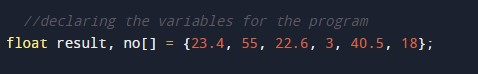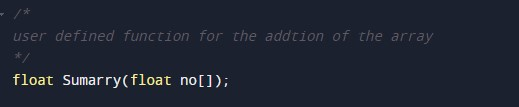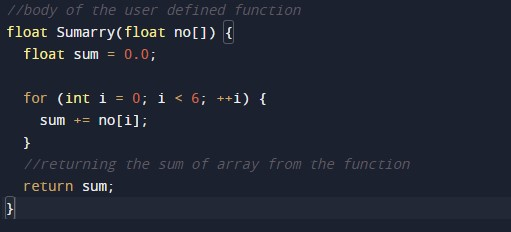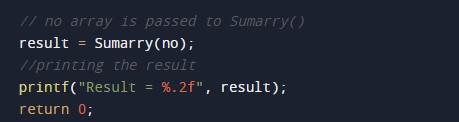C Program to Pass Array to Function

In this tutorial you will learn about the C Program to Pass Array to Function and its application with practical example.

C Program to Pass Array to Function

In this tutorial, we will learn to create a C program that will Pass Array to Function in C programming.

Prerequisites

Before starting with this tutorial we assume that you are best aware of the following C programming topics:

• Operators in C Programming.
• Basic Input and Output function in C Programming.
• Basic C programming.
• For loop in c programming.
• String functions of c programming.

Program to Pass Array to Function

As we all know the array is a collection of similar data types. In an array, only one variable is declared which can store multiple values. First, we will declare the input array for the program.

Then we will pass that array to a function for the calculation.

Then we will add the elements of using the for a loop.

With the help of this program, we can Pass Array to Function.

Output:-In the above program, we have first initialized the required variable.• no[] = it will hold the float value array.
• result = it will hold the float value.
• sum = it will hold the float value.
• i = it will hold the integer value for the loop control.

User-defined function declaration for the addition of array.Body of the user-defined function.Printing the output.In this tutorial we have learn about the C Program to Pass Array to Function and its application with practical example. I hope you will like this tutorial.